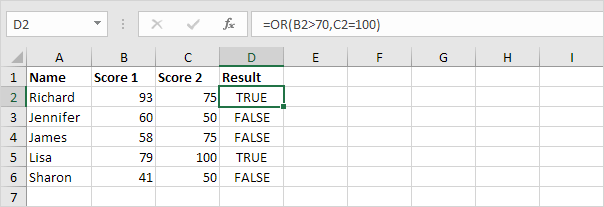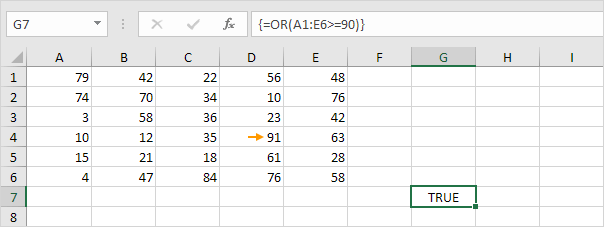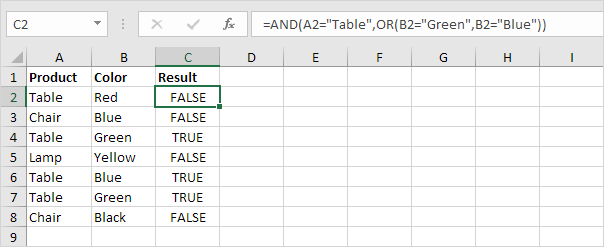# OR function

The OR function in Excel returns TRUE if any of the conditions are true and returns FALSE if all conditions are false. Combine the OR function with other functions and become an Excel expert.

1. For example, take a look at the OR function in cell D2 below.Explanation: the OR function returns TRUE if the first score is greater than 70 or the second score is equal to 100, else it returns FALSE.

2. Combine the OR function with the IF function.Explanation: the OR function returns TRUE or FALSE (see previous example). If TRUE, the IF function returns Pass, if FALSE, the IF function returns Fail.

3. For example, take a look at the OR function in cell E2 below.Explanation: the OR function returns TRUE if at least one score is greater than or equal to 90, else it returns FALSE. The OR function can test up to 255 conditions. The only way for the OR function to return FALSE is if all the conditions are FALSE (row 6).

4. The array formula below uses the OR function to return TRUE if any cell in the range A1:E6 is greater than or equal to 90.Explanation: finish an array formula by pressing CTRL + SHIFT + ENTER. Excel adds the curly braces {}. The value in cell D4 is greater than or equal to 90. As a result, the array formula returns TRUE.

5. Combine the OR function with the AND function.Explanation: the AND Function returns TRUE if all conditions are true and returns FALSE if any of the conditions are false. The AND function above has two arguments separated by a comma (Table, Green or Blue). The AND function returns TRUE if Product equals “Table” and Color equals “Green” or “Blue”.

6. Combine the OR function with the WEEKDAY function.Explanation: the WEEKDAY function in Excel returns a number from 1 (Sunday) to 7 (Saturday) representing the day of the week of a date. As a result, the OR function returns TRUE if the date falls on a weekend.

7. Don’t confuse the OR function with the XOR (Exclusive OR) function.Explanation: the XOR function returns TRUE if an odd number of conditions are true, and FALSE otherwise. With two conditions (see example above) the XOR function returns TRUE if one condition is true (row 2) and returns FALSE if both conditions are false (row 3, 4 and 6) or both conditions are true! (row 5).

Previous articleLocate Maximum Value in Excel
Next articlePercentage Formula in Excel – Easy Calculations